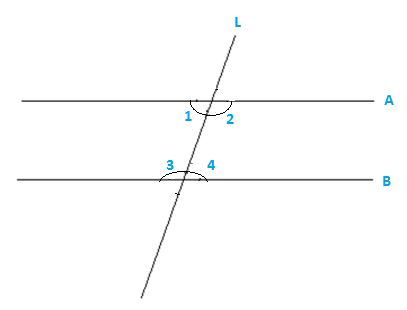Newbie

# . Does Euclid’s fifth postulate imply the existence of parallel lines? Explain. Q.2

• 0

How i solve this tricky question of ncert class 9th chapter of introduction to euclid’s geometry . Give me the best solution of exercise 5.2 question number 2 . Find the best and simplest solution of this tricky question give me the easiest method of this question.Does Euclid’s fifth postulate imply the existence of parallel lines? Explain.

Share